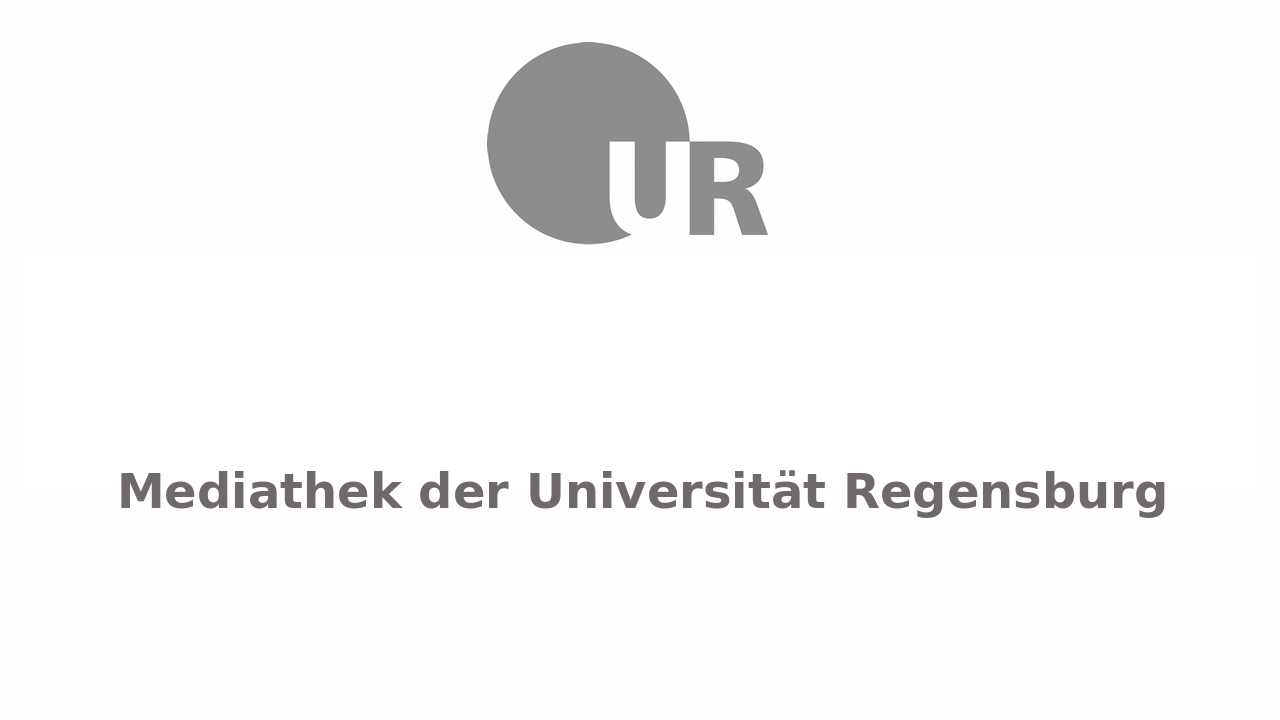# Kanal

## Quantum Computing Summer 2020Christoph Lehner
##### 01) Qubits, gates, measurementsChristoph Lehner
##### 02) Circuits, Bell state, simulator, many-qubit gatesChristoph Lehner
##### 03) Quantum parallelism, Deutsch Josza algorithm (I)Christoph Lehner
##### 04) Deutsch Josza (II), Grover (I)Christoph Lehner
##### 05) Grover (II), arithmetic gates, ancilla and garbage qubits, uncomputingChristoph LehnerChristoph Lehner
##### 07) Quantum Fourier transform (QFT), addition using QFT (I)Christoph Lehner
##### 08) Addition using QFT (II), phase estimation (I)Christoph Lehner
##### 09) Phase estimation (II)Christoph Lehner
##### 10) Order finding (I), arithmetic gates modulo n (I)Christoph Lehner
##### 11) Order finding (II), arithmetic gates modulo n (II), Shor's algorithm (I)Christoph Lehner
##### 12) Shor's algorithm (II), RSA encryption (I)Christoph Lehner
##### 13) RSA encryption (II), real quantum computing, characteristics of real quantum computers (I)Christoph Lehner
##### 14) Characteristics of real quantum computers (II), example of hardware implementation, Bell state on IBM systemChristoph Lehner
##### 15) Formal treatment of quantum noise; principal system and environment; mixed states, density matrix, and quantum operations (I)Christoph Lehner
##### 16) Mixed states, density matrix, and quantum operations (II), noise channels (I)Christoph Lehner
##### 17) Noise channels (II), fidelity and trace distance, simulating quantum noiseChristoph Lehner
##### 18) Quantum error correction, three-qubit bit flip codeChristoph Lehner
##### 19) The three-qubit phase-flip code, the 9-qubit Shor codeChristoph Lehner
##### 20) Stabilizer codes (I)Christoph Lehner
##### 21) Stabilizer codes (II), Gottesman-Knill theoremChristoph Lehner
##### 22) Fault tolerance, threshold theorem (I)Christoph Lehner
##### 23) Threshold theorem (II), fault tolerant operations, scientific quantum computingChristoph Lehner
##### 24) One-dimensional spin chain, one-dimensional free particle (I)Christoph Lehner